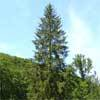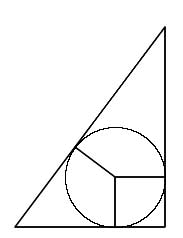You may also likeBaby Circle

A small circle fits between two touching circles so that all three circles touch each other and have a common tangent? What is the exact radius of the smallest circle?Logosquares

Ten squares form regular rings either with adjacent or opposite vertices touching. Calculate the inner and outer radii of the rings that surround the squares.Two Trees

Two trees 20 metres and 30 metres long, lean across a passageway between two vertical walls. They cross at a point 8 metres above the ground. What is the distance between the foot of the trees?

Incircles

Age 16 to 18Challenge Level(1) Show that the largest circle that fits inside a triangle whose sides have lengths 3, 4, 5 has radius 1. (2) Show that the largest circle that fits inside a triangle whose sides have lengths 5, 12, 13 has radius 2. (3) Can you find a right-angled triangle such that the largest circle that fits inside it has radius 3? Of course, one such triangle has sides of lengths 9,12,15 (which is obtained by scaling the 3,4,5 triangle by a factor 3) but can you find another?